# Subtraction up to 9

## NUMBERS AND OPERATIONSSubtraction is an arithmetic operation that is represented by a "minus" sign (-) and symbolizes the operation of removing objects from a collection.

Subtraction is anti-commutative

A modification in the order of its elements (minuend and subtrahend) will modify the sign of the answer; for example: 6 - 4 ≠ 4 - 6.

Subtraction is non-associative

When three or more numbers are subtracted, the order in which the subtraction is performed always influences; for example: 5 - (3 - 2) ≠ (5 - 3) - 2.

#### Get started with subtraction by understanding both the process of taking away and counting back on the number line to 9

What is subtraction for children and how do we teach it?

We are going to learn to solve subtractions with the natural numbers from 0 to 9 using as strategies the counting units and the subtraction of the elements of a set . Also, enjoy the explanations that accompany the video lesson that we propose.

SPANISHENGLISHTo understand correctly what a subtraction is, we provide you with educational worksheets where you have to count the number of elements in each set (either mentally or by hand) and check the result obtained when we remove or extract a certain amount:Therefore, we can say that "subtract" consists of removing, reducing or separating a part of the elements from an initial collection.#### Interpret the meaning of the subtraction signs (- e =) with natural numbers up to 9

What do the symbols "-" and "=" mean in a subtraction?

From the 15th century, the current mathematical signs that represent the subtraction operation began to be used in Europe:

- The symbol "-" is read "minus", "less" or "subtracted from" and is always inserted between the elements that we have to subtract.

Example: The subtraction sentence 6 - 2 is read "six minus two"

- The symbol "=" is read "equals", "equal", "is" or "are" and represents the result that we obtain after reducing, separating or extracting the elements of a total set into a new set.

Example: The subtraction equation 6 - 2 = 4 is read "six minus two equals four"

SPANISHENGLISH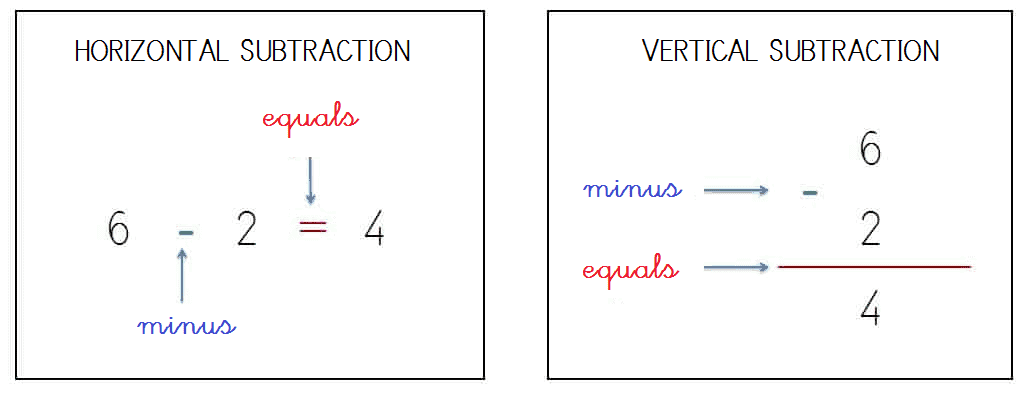We present you some files in PDF format where you have to count the elements of an initial collection and remove a part (also known as subtraction by crossing out) by demonstrating the equivalency between subtracting objects or subtracting numbers directly:#### Know the elements of subtraction and their position with natural numbers up to 9

What is the "minuend", the "subtrahend" and the "difference" in a subtraction?

Once we have recognized and interpreted the meaning of the mathematical symbols "-" e "=", we will explain in detail what the subtraction terms are:

- Minuend: Represents the total collection or initial set of elements.

- Subtrahend: Symbolizes the number or quantity of elements in which the minuend will be reduced.

- Difference or Rest: It corresponds to the unique set formed by separating or reducing the amount of the subtrahend on the minuend.

SPANISHENGLISH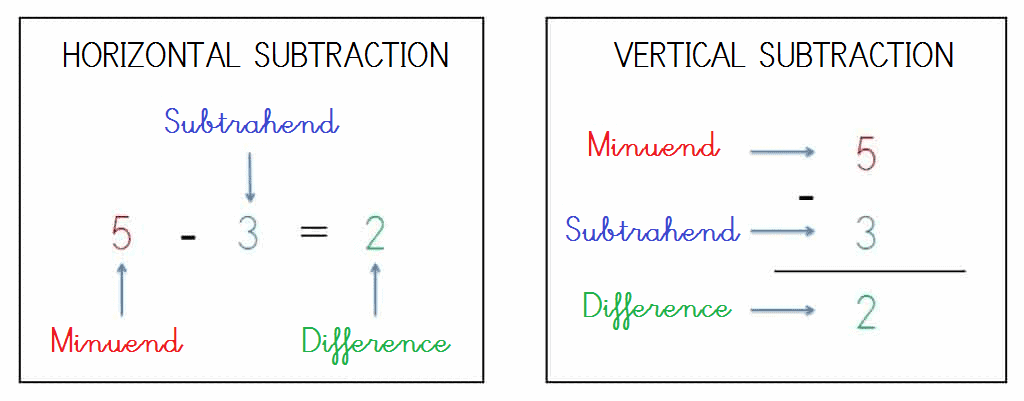#### Properly place the subtraction terms in natural numbers up to 9

Next you will learn to construct vertical subtractions placing the symbols "-" e "=" and each of its terms (minuend, subtrahend and difference) in the corresponding place practicing with the different printable educational worksheets that we have produced.

SPANISHENGLISH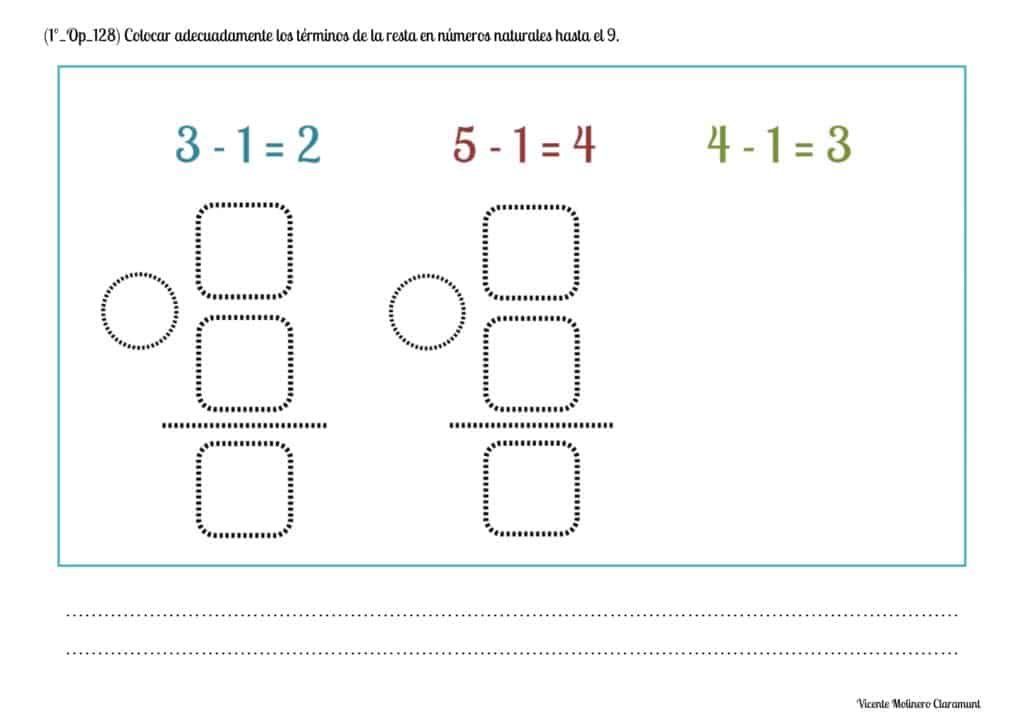#### Subtracting numbers vertically (with the help of the number line) with natural numbers up to 9

Practice the subtraction without regrouping (or without borrowing) vertically (in columns) with the numbers from 0 to 9 using the number line from the exercises that we propose.

SPANISHENGLISH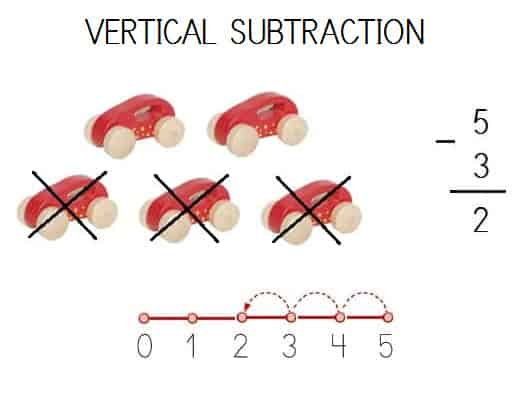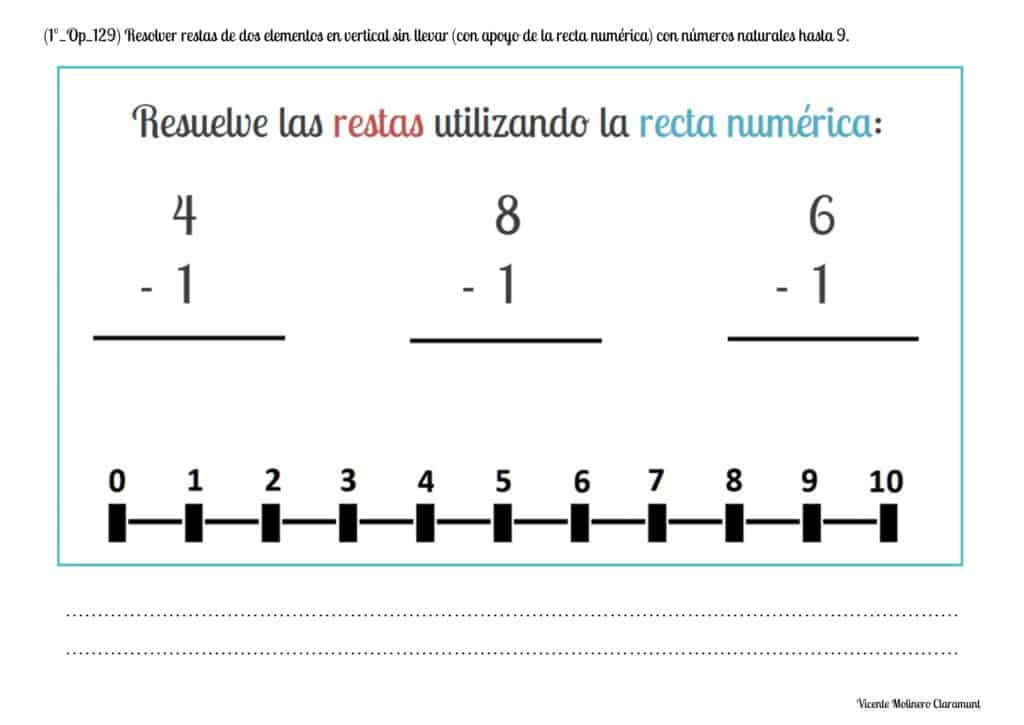#### Subtracting numbers vertically (without the help of the number line) with natural numbers up to 9

We provide you with a series of exercises so that you can continue practicing subtraction no borrowing (or no regrouping) vertically with the numbers from 0 to 9... Remember that you can help with fingers!

SPANISHENGLISH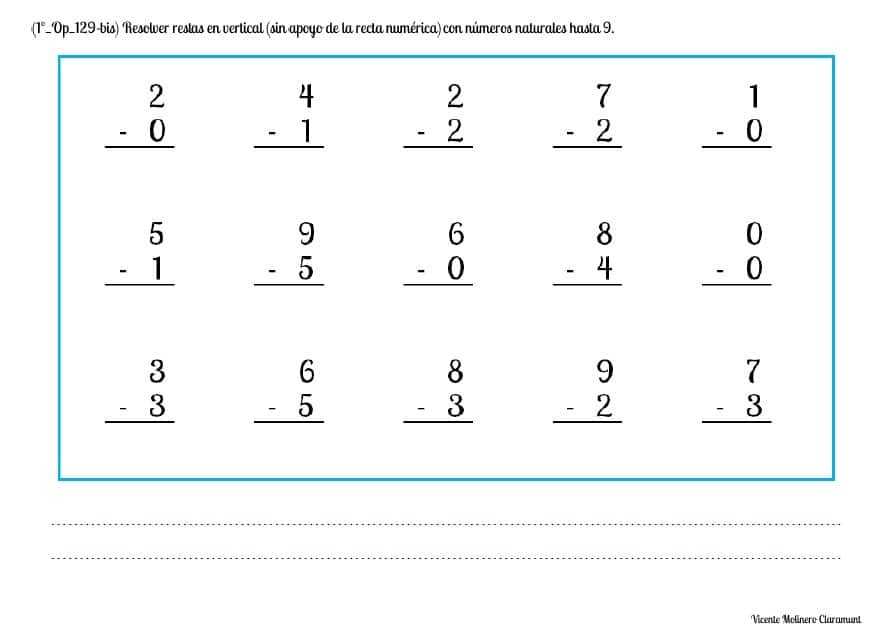#### Subtracting numbers horizontally (with the help of the number line) with natural numbers up to 9

Aprende a resolver restas horizontales sin llevar con números naturales del 0 al 9 con nuestros ejercicios didácticos partiendo del número mayor y, seguidamente, dando saltos descendentes sobre un recta numérica (ya sea real o imaginaria).

SPANISHENGLISH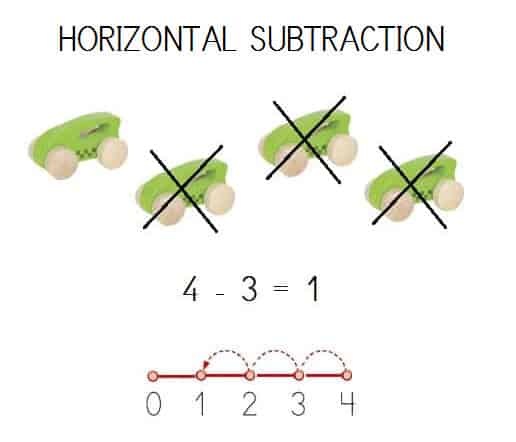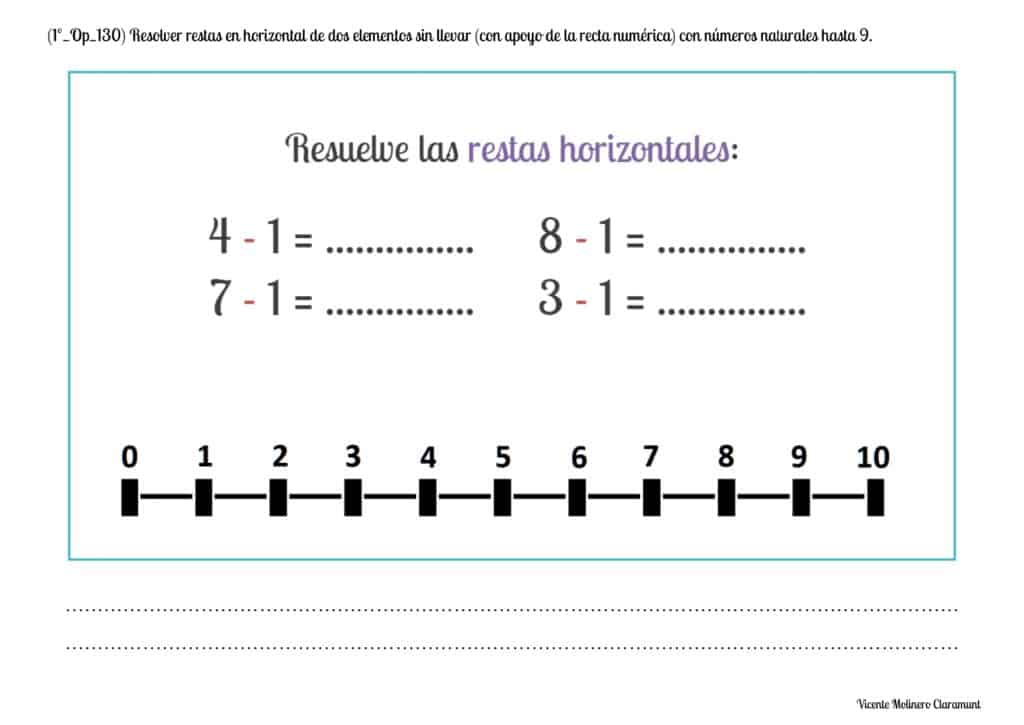#### Solve subtractions of three elements vertically without regrouping with whole numbers up to 9

Did you know that you can subtract three numbers? We explain how to solve this type of subtraction. Initially, you must subtract the first pair of numbers (in this case, 8 - 3 = 5) and then subtract the difference obtained over the third number (5 - 4 = 1). Now you try it!

SPANISHENGLISH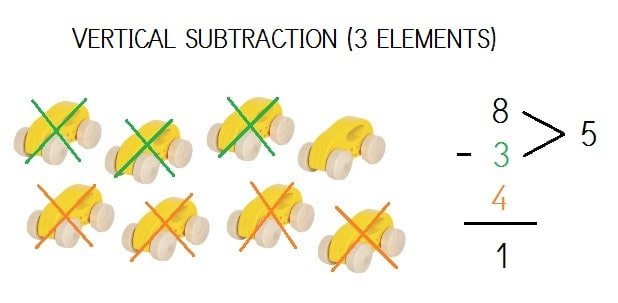#### Mentally solve simple subtraction facts with natural numbers up to 9

Show your logical-mathematical skills by solving our exercises with hidden or missing whole numbers and using mental calculation (also known as "mental math").#### Solve subtraction word problems without regrouping with whole numbers up to 9

Do you know how to solve a subtraction word problem easily?

SPANISHENGLISHWe explain step by step how to solve any word problem using subtraction:

1) Read the math problem very well (as many times as you need) until you make sure that you have understood it correctly.

2) Interpret what the problem asks of us, that is, understand where we want to go and what we want to achieve.

3) Identify, circle and / or underline the data of the problem and the question that it proposes to us (if there is one).

4) Decide how to use the data of the problem and which operation (or operations) will be the most appropriate to solve it (in this case, the subtraction).

5) Solve the operation (or operations) of subtraction and review the calculations to check the result again (auto-correction).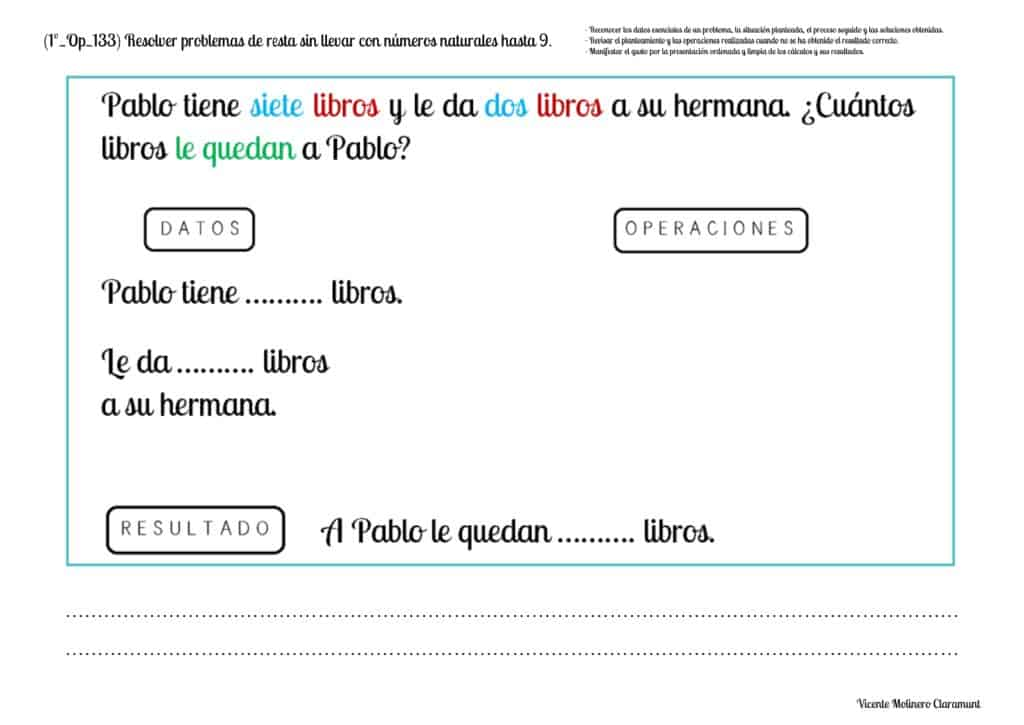Let's color!

We have selected dozens of Pinterest activities for you to practice coloring the basic addition and subtraction facts.

Open your case, take out the colored pencils and show the great artist in you!

Select more exercises, worksheets and activities of Mathematics for each of the 4 learning blocks of the Educational Curriculum ("Numbers and Operations", "Quantities and Measurements", "Geometric and Spatial Reasoning" and "Data Analysis and Probability") aimed at improving the logical-mathematical competencies and skills that are developed throughout "Grade 1" or "Year 2":